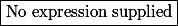# Matematické Fórum

Nevíte-li si rady s jakýmkoliv matematickým problémem, toto místo je pro vás jako dělané.

Nástěnka
!! 17.06.2018 (Jel.) Khanova škola zve nadšence ke spolupráci na překladech návodů pro učitele a rodiče.
! 04.11.2016 (Jel.) Čtete, prosím, před vložení dotazu, děkuji!
17.01.2016 (Jel.) Rok 2016 s novými a novějšími krystaly od kolegy Pavla!
17.01.2016 (Jel.) Nabídka knih z oborů matematiky, fyziky, chemie
23.10.2013 (Jel.) Zkuste před zadáním dotazu použít některý z online-nástrojů, konzultovat použití můžete v sekci CAS.

Nejste přihlášen(a). Přihlásit

## #1 09. 03. 2019 09:25

stuart clarkPříspěvky: 971
Reputace:

### function

Let$g(x)$ is defined for$x>-1$ and has a continuous derivatives. If$g(x)$ satisfies$g(0)=1,g'(0)=0$ and$(1+g(x))g''(x)=1+x.$ Then maximum value of$g'(2)$ is

and also proving$x=0,g(x)$ has minima and function$g(x)$ is concave upwards

Offline

## #2 17. 03. 2019 12:50

laszky
Příspěvky: 1529
Škola: MFF UK, FJFI CVUT
Reputace:   121

### Re: function

↑ stuart clark:

Hi, numerical solution gives$g'(2)\approx1.594$

Online

## #3 17. 03. 2019 16:03

BatiPříspěvky: 2205
Reputace:   172

### Re: function

Hi ↑ laszky:,
the numerical result is useless unless you provide an error estimate and the method that you used.

Offline

## #4 17. 03. 2019 16:32

laszky
Příspěvky: 1529
Škola: MFF UK, FJFI CVUT
Reputace:   121

### Re: function

↑ Bati:

You have probably never seen the weather forcast :D  Is it useless?

Online

## #5 17. 03. 2019 16:45

jardofprPříspěvky: 1188
Reputace:   79

### Re: function

hey guys,

to follow the comment from ↑ Bati:,

I would add that I believe that also proving global uniqueness for solution on interval$(-1,\infty)$

is necessary to give the numerical solution some weight, assuming some numerical method for ODE was used

in case of ODE with more solutions, numerical approximation of one of the solutions
can be not anywhere close to full picture

(convexity and minimum of the function is very easy in this problem,
I'm just getting curious about the requested maximum estimate)

Offline

## #6 17. 03. 2019 18:29

BatiPříspěvky: 2205
Reputace:   172

### Re: function

↑ laszky:
So you basically say that your result is as reliable as a weather forecast... and yes, a bad forecast is pretty useless

↑ jardofpr:
Good point

Offline

## #7 23. 03. 2019 13:18

stuart clarkPříspěvky: 971
Reputace:

### Re: function

↑ jardofpr:

How can i find convexity and minimum of function. Thanks

Offline

## #8 23. 03. 2019 17:03

jardofprPříspěvky: 1188
Reputace:   79

### Re: function

hi ↑ stuart clark:

There is$(1+g(0))g''(0) = 1$ therefore$g''(0) = \frac{1}{2}$

together with$g'(0)=0$ it says that the function has at least local minimum in$x=0$.

Furthermore$g$ is defined for$x>-1$ so there is$(1+g(x))g''(x)>0$ on the domain of the function

(note that inequality is sharp, never equality)

so in each$x$ expressions$(1+g(x))$  and$g''(x)$  are either both strictly positive or both strictly negative.

As$g''(0)>0$  and given$g$ and its derivatives are  continuous,$g''(x)$ stays always positive

and therefore$g$ is concave upwards = convex.

This fact also says that$g'(x)$ is strictly increasing,  and together with$g'(0)=0$ this gives$g'(0)<0\,,\,\forall x\in(-1,0)$   and$g'(0)>0\,,\,\forall x\in (0,\infty)$, which makes the minimum in$x=0$ global.

To the last unanswered question I was yet able to find out that$g'(2) < 2$ by analytical approach
but this is for sure not maximum as it cannot be reached.

Offline

## #9 25. 03. 2019 12:37

stuart clarkPříspěvky: 971
Reputace:

### Re: function

Thanks ↑ jardofpr:

Offline